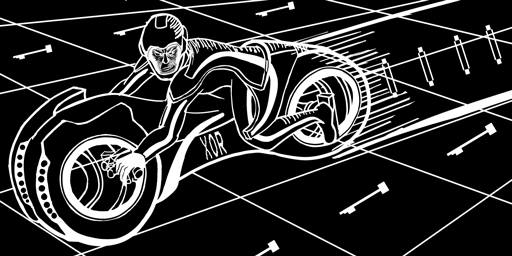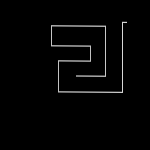# 219 bytes tron

With some coworkers, we challenged each other to write the smallest possible game of tron in javascript (an exercise known as javascript golfing).

This page explains our final version (219 bytes). We initially worked alone but then exchanged ideas and tricks, so erling & mathewsb deserve most of the credits!

our code was originally 226 bytes, but "Cosmologicon" pointed out a way to save three whole bytes, bringing us to 223 bytes.

With p01, we then came up with a way to save another 11 bytes (making the game 212 bytes). He also suggested keeping track of score, which takes 9 bytes but is totally worth it!

skrounge found a way to save 2 more bytes, bringing the game to 219 bytes.

Danny Loo sent me this piece of art. It does a nice job depicting what's going on, read on...We started with some basic rules:
1. the tron must start in the center, facing any direction.
2. the game controls must be 'i', 'j', 'k', 'l'.
3. when the tron hits it's trail or an edge, "game over" must be shown to the user.
4. the code need only run on Chrome 17.

Unlike some js size optimization competitions, we did not use a shim. We took into account the entire page's size. This forced us to explore novel tricks.

My coworkers represented the board as ascii-art (which resulted in shorter code but an unplayable game). I preferred to keep the game nicer and use a <canvas> tag.```<body id=b onkeyup=e=event onload=
z=c.getContext('2d');
z.fillRect(s=0,0,n=150,x=11325);
setInterval("
0<x%n
&x<n*n
&(z[x+=[1,-n,-1,n][e.which&3]]^=1)
?z.clearRect(x%n,x/n,1,1,s++)
:b.innerHTML='game⬜over:'+s
",9)
><canvas id=c>
```

The entire code, with only a few extra newlines for readability.

```<body id=b onkeyup=e=event onload=
z=c.getContext('2d');
z.fillRect(s=0,0,n=150,x=11325);
setInterval("
0<x%n
&x<n*n
&(z[x+=[1,-n,-1,n][e.which&3]]^=1)
?z.clearRect(x%n,x/n,1,1,s++)
:b.innerHTML='game⬜over:'+s
",9)
><canvas id=c>
```

By assigning an `id` to the body, we are going to be able to write `b.innerHTML="game over"`, instead of having to write `document.body.innerHTML="game over"`*. Saves us 7 bytes.

We can also drop the quotes around the attribute, since browsers are able to fix that for us.

* In Firefox, this only works in Quirks Mode (which is enabled since there isn't a doctype in the demo).

```<body id=b onkeyup=e=event onload=
z=c.getContext('2d');
z.fillRect(s=0,0,n=150,x=11325);
setInterval("
0<x%n
&x<n*n
&(z[x+=[1,-n,-1,n][e.which&3]]^=1)
?z.clearRect(x%n,x/n,1,1,s++)
:b.innerHTML='game⬜over:'+s
",9)
><canvas id=c>
```

We use `onkeyup` instead of `onkeydown`, since it saves 2 bytes. The game feels a little different, but who cares?

`e` is going to contain the keyboard event. Implies the game is going to spew js errors until the first key is released. It also means the game doesn't start until you press the first key. This can be improved, but requires 2 extra bytes.

```<body id=b onkeyup=e=event onload=
z=c.getContext('2d');
z.fillRect(s=0,0,n=150,x=11325);
setInterval("
0<x%n
&x<n*n
&(z[x+=[1,-n,-1,n][e.which&3]]^=1)
?z.clearRect(x%n,x/n,1,1,s++)
:b.innerHTML='game⬜over:'+s
",9)
><canvas id=c>
```

We put our main code in a `onload=`, since it saves us the <script> and </script> tags (saves 10 bytes). We are not going to use quotes, so there is a set of characters we can't use in the code (as it would end the attribute). The set of forbidden characters includes >, space, tab.

Note: the `<script>` tag always requires a closing tag.

```<body id=b onkeyup=e=event onload=
z=c.getContext('2d');
z.fillRect(s=0,0,n=150,x=11325);
setInterval("
0<x%n
&x<n*n
&(z[x+=[1,-n,-1,n][e.which&3]]^=1)
?z.clearRect(x%n,x/n,1,1,s++)
:b.innerHTML='game⬜over:'+s
",9)
><canvas id=c>
```

`z` is going to contain the context for the canvas. There is no way to avoid this expensive expression. We are however also going to use `z` to store our grid to detect collisions. JavaScript lets you access properties on objects using the array [] syntax, and everything works out as long as you don't need to call array functions (i.e. we can't do `z.join(…);`).

Reusing `z` to store our grid saves 2 to 5 bytes, depending on how things are done. The grid is going to be a single dimension, n*n grid.

Note: we tried using the canvas to detect collisions. Accessing the pixel values of a canvas turned out to require lots of bytes.

```<body id=b onkeyup=e=event onload=
z=c.getContext('2d');
z.fillRect(s=0,0,n=150,x=11325);
setInterval("
0<x%n
&x<n*n
&(z[x+=[1,-n,-1,n][e.which&3]]^=1)
?z.clearRect(x%n,x/n,1,1,s++)
:b.innerHTML='game⬜over:'+s
",9)
><canvas id=c>
```

Draws the black background.

By default, an empty canvas has a black foreground color, white background color and is 300x150 pixels wide.

We also initialize 3 variables, `n` is set to 150 (which is going to be our arena size).

`x` is going to be the tron's position, and is set to 11325 (11325=75*75+75=center of the grid).

`s` is going to keep track of the score and is set to 0.

We are effectively drawing a 150x11325 box, but the game is going to look & feel 150x150.

Simultaneously calling a function and setting a variable is a very common trick in js golf and saves lots of bytes.

```<body id=b onkeyup=e=event onload=
z=c.getContext('2d');
z.fillRect(s=0,0,n=150,x=11325);
setInterval("
0<x%n
&x<n*n
&(z[x+=[1,-n,-1,n][e.which&3]]^=1)
?z.clearRect(x%n,x/n,1,1,s++)
:b.innerHTML='game⬜over:'+s
",9)
><canvas id=c>
```

`setInterval` causes our code to get called every 9ms. The code inside `setInterval` is the main game loop. It updates the tron's position and detects collisions.

The code is written as a string, as it's shorter than writing `function(){…}` (saves 10 bytes). This implies the main loop cannot use the ", >, space and tab characters.

```<body id=b onkeyup=e=event onload=
z=c.getContext('2d');
z.fillRect(s=0,0,n=150,x=11325);
setInterval("
0<x%n
&x<n*n
&(z[x+=[1,-n,-1,n][e.which&3]]^=1)
?z.clearRect(x%n,x/n,1,1,s++)
:b.innerHTML='game⬜over:'+s
",9)
><canvas id=c>
```

Checks if we are within the game boundary. We can't use the `>` operator (we are inside an attribute), but `<` works.

• If the tron hits the left edge, `x%n` will return `0`.

• If the tron hits the right edge, `x` will wrap around and `x%n` will return `0`.

• If the tron hits the top edge, `x` will become negative and `x%n` will return a negative value.

• If the tron hits the bottom edge, `x` will be larger than `n*n`.

skrounge pointed out that we can use the `&` (binary and) instead of `&&` (logical and) since both sides of the operator are boolean values.

```<body id=b onkeyup=e=event onload=
z=c.getContext('2d');
z.fillRect(s=0,0,n=150,x=11325);
setInterval("
0<x%n
&x<n*n
&(z[x+=[1,-n,-1,n][e.which&3]]^=1)
?z.clearRect(x%n,x/n,1,1,s++)
:b.innerHTML='game⬜over:'+s
",9)
><canvas id=c>
```

Updates the variable `x` based on the value of `e` (remember, onkeyup saves the event in the `e` variable).

The grid is a single dimension, going up or down requires us to add or subtract `n` units.

Besides being placed in a cross shape on the keyboard, 'i', 'j', 'k', 'l' are also consecutive letters. We convert the key code into an index by simply doing `&3` (notice how `e.which` is shorter than `e.keyCode` by 2 bytes).

Note: hitting any other key will also move the tron in various directions. Filtering all other keys requires 4 more bytes.

```<body id=b onkeyup=e=event onload=
z=c.getContext('2d');
z.fillRect(s=0,0,n=150,x=11325);
setInterval("
0<x%n
&x<n*n
&(z[x+=[1,-n,-1,n][e.which&3]]^=1)
?z.clearRect(x%n,x/n,1,1,s++)
:b.innerHTML='game⬜over:'+s
",9)
><canvas id=c>
```

This expression is one of the nicest parts of the code. There's a lot going on here:

• The grid is never initialized, so it starts out with all the values set to `undefined`.

• The `^=` (bitwise xor assignment) operator lets us update the grid while checking for a collision:

• If the value in the grid has never been visited, we set the grid value to `1` and return `1`.

• If the value in the grid has been visited, we return `0`.

```<body id=b onkeyup=e=event onload=
z=c.getContext('2d');
z.fillRect(s=0,0,n=150,x=11325);
setInterval("
0<x%n
&x<n*n
&(z[x+=[1,-n,-1,n][e.which&3]]^=1)
?z.clearRect(x%n,x/n,1,1,s++)
:b.innerHTML='game⬜over:'+s
",9)
><canvas id=c>
```

Using the ternary `?:` operator, we draw a white pixel at the tron's position when the game has not ended. Since our grid and `x` are a single dimension, we need to use `/` and `%` operators to get each coordinate value.

We also increment the score. Another common trick to save bytes is to add extra parameters to function calls.

```<body id=b onkeyup=e=event onload=
z=c.getContext('2d');
z.fillRect(s=0,0,n=150,x=11325);
setInterval("
0<x%n
&x<n*n
&(z[x+=[1,-n,-1,n][e.which&3]]^=1)
?z.clearRect(x%n,x/n,1,1,s++)
:b.innerHTML='game⬜over:'+s
",9)
><canvas id=c>
```

We replace the page's content with "game over" and the score when a collision occurs.

Since we are inside an attribute we cannot use a space character. We instead use U+00A0 (non breaking space) and shown here as ⬜. In iso88591 this only takes one byte. The browser auto detects iso88591 if we don't serve the page with another content type.

Note: the game still exists in memory and is still running.

```<body id=b onkeyup=e=event onload=
z=c.getContext('2d');
z.fillRect(s=0,0,n=150,x=11325);
setInterval("
0<x%n
&x<n*n
&(z[x+=[1,-n,-1,n][e.which&3]]^=1)
?z.clearRect(x%n,x/n,1,1,s++)
:b.innerHTML='game⬜over:'+s
",9)
><canvas id=c>
```

Defines the canvas. We use the same trick of setting an `id` on canvas, which reappears in the global space.

note: we removed any unnecessary tags, like `<html>`, `<head>`, `</head>`, `</canvas>`, `</body>`, `</head>` and `</html>`.